#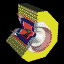The Upsilon System

The Upsilon system is the bound state of a b quark and anti b quark, bound together somewhat analogously to the electron and proton of a hydrogen atom. Various excited states (resonances) of this system can be created by tuning the accelerator energy, as shown by this plot of the rate of particle production as a function of energy in the region of the various Upsilon states (as measured by the CUSB detector):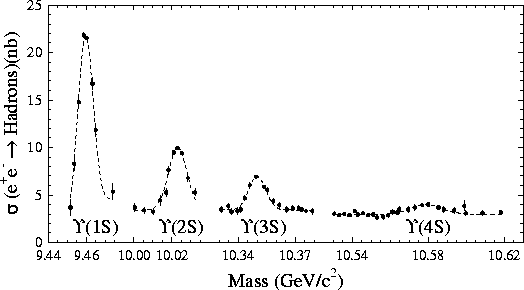At the first three resonances, the Upsilon system can only decay by the b quark and anti b quark annihilating, as shown in the decay diagrams (a)-(c):At the fourth resonance, called the Upsilon(4S), there is enough energy in the excited stated to create a light quark/anti-quark pair, producing a pair of B mesons, as shown in diagram (d) above. Once produced, B mesons can decay via any one of the processes illustrated here: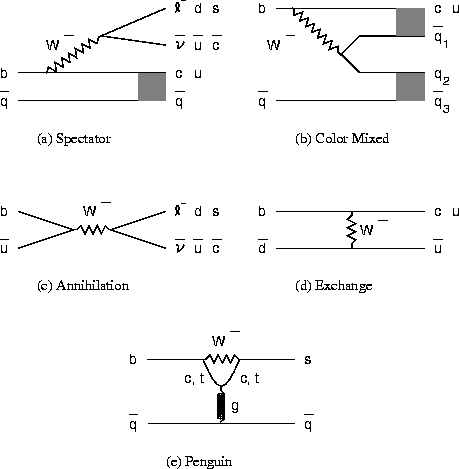So far, experiments studying the Upsilon system and the B mesons produced there have confirmed the Standard Model, disproved many competing models, and placed new limits on the values of the KM-angles and the mass of the t quark.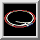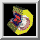dsr@mail.lns.cornell.edu
\$Id: upsilon.html,v 1.4 2001/07/19 16:18:28 dsr Exp \$